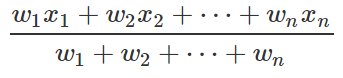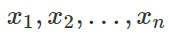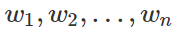# Weighted Average Calculator

By Mateusz Mucha and Hanna Pamuła, PhD candidate
Last updated: Jul 15, 2020

To understand how a weighted average calculator works, you must first understand what a weighted average is. Weighted average has nothing to do with weight conversion, but people sometimes confuse these two concepts. The typical average, or mean, is when all values are added and divided by the total number of values. This can be computed using our average calculator, by hand or by using a hand-held calculator, since all the values have equal weights.

But what happens when values have different weights, which means that they're not equally important? Below you will see how to calculate the weighted mean using the weighted average formula. Also, you'll find examples where the weighted average method may be used - like e.g. calculation of the GPA, average grade or your final grade.

## Let's start from the beginning: what is a weighted average?

Weighted average (weighted arithmetic mean) is a concept similar to standard arithmetic mean (called simply the average), but in the weighted average not all elements are contributing equally to the final result. We can say that some values are more important than the others, so they are multiplied by a coefficient called the weight.

For example, during your studies you may encounter the situation where the grade from an exam is two times more important than the grade from a quiz - and that's exactly what we call the weighted average method. To define it in a more mathematical way, we can write the weighted average formula as:whereare our numbers, andare our weights - the importance of the numbers in averaging.

So, having A from an exam and C from a quiz, you'd get B as a standard average, but assuming that the exam is two times more important, you should get a B+.

## How to calculate a weighted average

One type of average which is typically weighted is a grade point average. As the calculation of GPA may sometimes be tricky, we've created two dedicated tools: the high school GPA and the college GPA calculator - have you checked them yet?

Let's find out how to calculate a weighted average - the easiest way is to look at the simple example:

Suppose a student has two four-credit classes, a three-credit class, and a two-credit class. Assume that the grades of the courses are as follows:

• A for a four-credit class,
• B for the other four credit class,
• A for the three credit class,
• C+ for the two credit class.

Then, we need to translate the letter grades into numerical values. Most schools in the US use a so-called 4.0 GPA scale, which is a 4 point grading scale. The table below shows a typical letter grade/GPA conversion system:

Letter Grade Percentile 4.0 scale +4.0 scale
A+ 97-100 4 4.3
A 93-96 4 4
A- 90-92 3.7 3.7
B+ 87-89 3.3 3.3
B 83-86 3 3
B- 80-82 2.7 2.7
C+ 77-79 2.3 2.3
C 73-76 2 2
C- 70-72 1.7 1.7
D+ 67-69 1.3 1.3
D 65-66 1 1
F Below 65 0 0

So from the table we know that A = 4.0, B = 3.0 and C+ = 2.3. Now that we have all the information, we can have a look at how to calculate the GPA using a weighted average method:

1. Sum the number of credits. `4 + 4 + 3 + 2 = 13`, that was a really easy step.
2. Take the value assigned to the grade and multiply by the number of credits. In our case, it will be:
• A * 4 credits = `4.0 * 4 = 16`
• B * 4 credits = `3.0 * 4 = 12`
• A * 3 credits = `4.0 * 3 = 12`
• C * 2 credits = `2.3 * 2 = 4.6`
1. Add all the values. `16 + 12 + 12 + 4.6 = 44.6`
2. Divide the sum by the total number of credits. So for our example it's equal to `44.6/13 = 3.43`

The whole weighted average formula may be written as:

`(4 * 4 + 4 * 3 + 3 * 4 + 2.3 * 2) / (4 + 4 + 3 + 2) = 3.43`

Let's compare this result to an average that is not weighted. Then we don't take the credits into account, and we divide the sum of grades by its total number.

`(4 + 3 + 4 + 2.3) / 4 = 3.33`

Notice how the weighted average changed. Sometimes it may be a really significant difference - like a grade difference or even whether you pass or fail your course.

## Weighted average formula

Let's repeat what the weighted average formula looks like:But what does it mean? To figure out how to calculate a weighted average, we need to know the weight of each value. Typically, we present the weights in the form of a percentage, or (in statistics) a probability of occurrence. For example, let's suppose that exams, quizzes and homework assignments all contribute to the grade of a class. Each of the three exams is worth 25 percent of the grade, the quizzes are worth 15 percent and the homework assignments are worth 10 percent. To calculate the average you multiply the percentage by the grades and add together. If the test scores are 75, 90, 88, the quiz average is 70, and the homework grade is 86, the weighted average is as follows:

`(0.25 * 75 + 0.25 * 90 + 0.25 * 88 + 0.15 * 70 + 0.10 * 86) / 1 = 82.35`

Compare this to a non-weighted average of `(75 + 90 + 88 + 70 + 86) / 5 = 81.8`

In statistics, you will often encounter a discrete probability distribution which has values for x and their associated probabilities. Since the probabilities for each value of x will likely not all be the same, we can apply the weighted average formula. Simply multiply each x value by its probability of occurring and sum the values.

## Weighted vs. unweighted GPA for high school

A weighted average is often used to calculate the so-called weighted GPA. It's a term which rarely appears in the context of college GPA (although college GPA is computed using a weighted average method, with courses credits as weights), but is usually used for high school GPA. Let's have a closer look at this topic.

The first thing which needs to be emphasized: you need to be precise, what you want to take into account during weighting - credits, course difficulty or maybe both these factors?

Course difficulty is taken into account in most weighted GPA calculations. It rewards you for taking classes of a higher level by adding extra points to your grade. There are a couple of types of more demanding courses, which influence your weighted GPA score:

• AP Courses (Advanced Placement Courses) usually give you additional 1 point to your standard GPA score,
• IB Courses (International Baccalaureate Courses) are also rewarded with 1 extra point,
• Honors Courses most often give you additional 0.5 point (although you can find examples of schools where it's awarded with 1 point).

So, what are the options of weighing in High school GPA calculations? Let's define:

1. Unweighted GPA, as the GPA where we DON'T care about course difficulty

`````` a) and we DON'T care about course credits

`High School GPA = Σ grade value / Σ courses`

b) and we DO care about course credits

`High School GPA = Σ (grade value * credits) / Σ credits`
``````
2. Weighted GPA, as the GPA where we DO care about course difficulty

`````` a) and we DON'T care about course credits

`High School GPA = Σ (weighted grade value) / Σ courses`

b) and we DO care about course credits

`High School GPA = Σ (weighted grade value * credits)/ Σ credits`
``````

It may look a bit overwhelming, but let's have a look at a hypothetical results sheet, and everything should be clear:

Maths A
Physics B+
Physics lab C+
English A-

• 1 a) Unweighted GPA: we DON'T care about course difficulty and credits

All the courses have the same grade scale and credits, no matter the course difficulty. So our grades may be converted into numbers:

Maths A 4.0
Physics B+ 3.3
Physics lab C+ 2.3
English A- 3.7

Then, we can calculate the unweighted GPA as:

`Unweighted High School GPA = Σ grade value / Σ courses `

`= (4.0 + 3.3 + 2.3 + 3.7) / 4 = 13.3 / 4 = 3.325 ≈ 3.33`

Did you notice that it's a standard average? It's just summing all scores and dividing the result by the total number of observations (4 courses).

• 1 b) Unweighted GPA: we DON'T care about course difficulty, but we DO care about credits

The things are getting more complicated when we consider the credits for the courses. Some sources ignore the course's credits for unweighted GPA score, but others keep them. So, if your classes have some credits/points, you can calculate the weighted average of grades and credits (but still, it's not the thing we usually name the weighted GPA):

Maths 0.5 A 4.0
Physics 1 B+ 3.3
Physics lab 0.5 C+ 2.3
English 1 A- 3.7

Then, the GPA will be equal to:

`High School GPA = Σ (grade value * credits) / Σ credits `

` = (4.0 * 0.5 + 3.3 * 1 + 2.3 * 0.5 + 3.7 * 1) / (0.5 + 1 + 0.5 + 1)`

` = 10.15 / 3 = 3.38333... ≈ 3.38`

The courses with higher credits value have better marks in our example, so the overall GPA is also higher.

• 2 a) Weighted GPA: we DO care about course difficulty, and DON'T care about course credits

Depending on the course type, the letter grades are translated to different numerical values:

Letter Grade Percentile Regular GPA Honors GPA AP / IB / College Prep GPA
A+ 97-100 4 4.5 5
A 93-96 4 4.5 5
A- 90-92 3.7 4.2 4.7
B+ 87-89 3.3 3.8 4.3
B 83-86 3 3.5 4
B- 80-82 2.7 3.2 3.7
C+ 77-79 2.3 2.8 3.3
C 73-76 2 2.5 3
C- 70-72 1.7 2.2 2.7
D+ 67-69 1.3 1.8 2.3
D 65-66 1 1.5 2
F Below 65 0 0 0

Continuing with our example, now our four classes have the course type assigned:

Course Credits Grade Course Type GPA Scale
Maths 0.5 A Honors 4.5
Physics 1 B+ Regular 3.3
Physics lab 0.5 C+ Regular 2.3
English 1 A- AP 4.7

As two courses are not standard classes, they get extra points (A from Maths - 4.5 instead of 4.0, as it's an Honors course, A- from English - 4.7 instead of 3.7, as it's an AP course).

The formula for the calculation of weighted GPA is:

`Weighted High School GPA = Σ (weighted grade value) / Σ courses `

`= (4.5 + 3.3 + 2.3 + 4.7) / 4 = 14.8 / 4 = 3.7 `

where `weighted grade value` is a:

• `grade value + 0` for Regular courses
• `grade value + 0.5` for Honors courses
• `grade value + 1` for AP/IB/College Prep courses

So we omitted the courses credits, but we've taken into account the difficulty of the course. And finally, we have

• 2 b) Weighted GPA: we DO care about course difficulty and DO care about course credits

So if you're taking into account both credits and course difficulty, then the result is:

`Weighted High School GPA = Σ (weighted grade value * credits) / Σ credits`

`= (4.5 * 0.5 + 3.3 * 1 + 2.3 * 0.5 + 4.7 * 1) / (0.5 + 1 + 0.5 + 1) = 11.4 / 3 = 3.8`

That wasn't so hard, was it?

## Different averages: arithmetic, geometric, harmonic

Now that you understood what a weighted average is, let's compare different averages. We've prepared for you a table which sums up all the important information about four different means:

Arithmetic Mean Geometric Mean Harmonic Mean Weighted Mean (Weighted Arithmetic Mean)
Definition sum of observations divided by the total number of observations the 'n'th root product of 'n' observations the reciprocal of the arithmetic mean of the reciprocals of the given set of observations numbers multiplied by a weight (based on relative importance), summed, divided by the sum of weights
Examples:
4 and 9
A = (4 + 9) / 2 = 6.5 G = √(4 * 9) = √36 = 6 H = 2 / (1/4 + 1/9) ≈ 5.54 Additional info - weights.
w1 = 3 w2 = 1
W = (4 * 3 + 9 * 1) / 4 = 5.25
Applications many different fields, e.g. economics, physics (e.g. mean free path), biology, history, everyday life and health (e.g. mean arterial pressure) business (investment, CAGR),
math (rectangle area in terms of square side, analogically volume),
signal processing (spectral flatness, choosing an aspect ratio)
many situations involving rates and ratios in physics (e.g. average speed), averaging multiples in finance (such as the price–earnings ratio), geometry, chemistry, computer science education (GPA, final grades, average grades), finances (e.g. WACC - Weighted Average Cost of Capital)
Relationship Arithmetic mean ≥ Geometric mean ≥ Harmonic mean (for non-negative data)

General formulas for means look as follows:

• Arithmetic mean:• Geometric mean:• Harmonic mean:• Weighted meanMateusz Mucha and Hanna Pamuła, PhD candidate
Values
#1
#2
#3
#4
#5
#6
#7
#8
Weights
#1
#2
#3
#4
#5
#6
#7
#8
People also viewed…

### Exponential growth

The exponential growth calculator calculates the final value of some quantity, given its initial value, rate of growth and elapsed time.